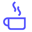a concise shot of dev knowledge
Become a Contributor
Concise shots of dev knowledge

RELATED TAGS

binary
search
algorithm
sorting

# What is a binary search?Edpresso Team

Binary search is an efficient method for locating an element in a sorted array that is similar to searching for a word in the dictionary. If the word to search starts with the letter $S$, one usually starts searching the dictionary around the half-way mark. Since it is commonly known that $S$ is located in the second half of the alphabet, it would not make sense to start searching for $S$ from the dictionary’s start.

## Algorithm

Just like in the dictionary example, when applying binary search to an array, we repeatedly divide it into individual search intervals. Initially, the original array is the search interval. If the value of the desired item is lesser than the item in​ the middle of the interval, then the search interval is the array with items smaller than the middle item. Similarly, if the desired item is greater than the item at the middle point, then the search interval is the array with items larger than the middle item.

This concept is illustrated in the slides below:

1 of 4

The time complexity of a binary search is $O(Log(n))$. The following code snippets demonstrate the recursive implementation of binary search:

#include <iostream>
using namespace std;

int binarySearch(int sample_arr[], int left, int right, int key) {

//Base Case: binary search executes as long right-most index is greater
// than left-most index.
if (right >= left) {
int mid = left + (right - left) / 2;

// if element is present at the middle return index.
if (sample_arr[mid] == key)
return mid;

// If element is smaller than mid, then
// it can only be present in left subarray
if (sample_arr[mid] > key)
return binarySearch(sample_arr, left, mid - 1, key);

// Else the element can only be present
// in right subarray
return binarySearch(sample_arr, mid + 1, right, key);

}

return -1;

}

int main() {

int sample_arr[] = {2, 5, 8, 12, 16, 23, 38, 56, 72, 91};
int arr_size =  sizeof(sample_arr) / sizeof(sample_arr);
int key = 23;

int result = binarySearch(sample_arr, 0, arr_size - 1, key);
(result == -1) ? printf("Element is not present in array")
: printf("Element is present at index %d",
result);

return 0;
}

RELATED TAGS

binary
search
algorithm
sorting
RELATED COURSES

View all Courses

Keep Exploring

Learn in-demand tech skills in half the time